Published

# Arduino-Based Security Alarm

This security alarm is an easy-to-use, efficient product that can function anytime, and anywhere.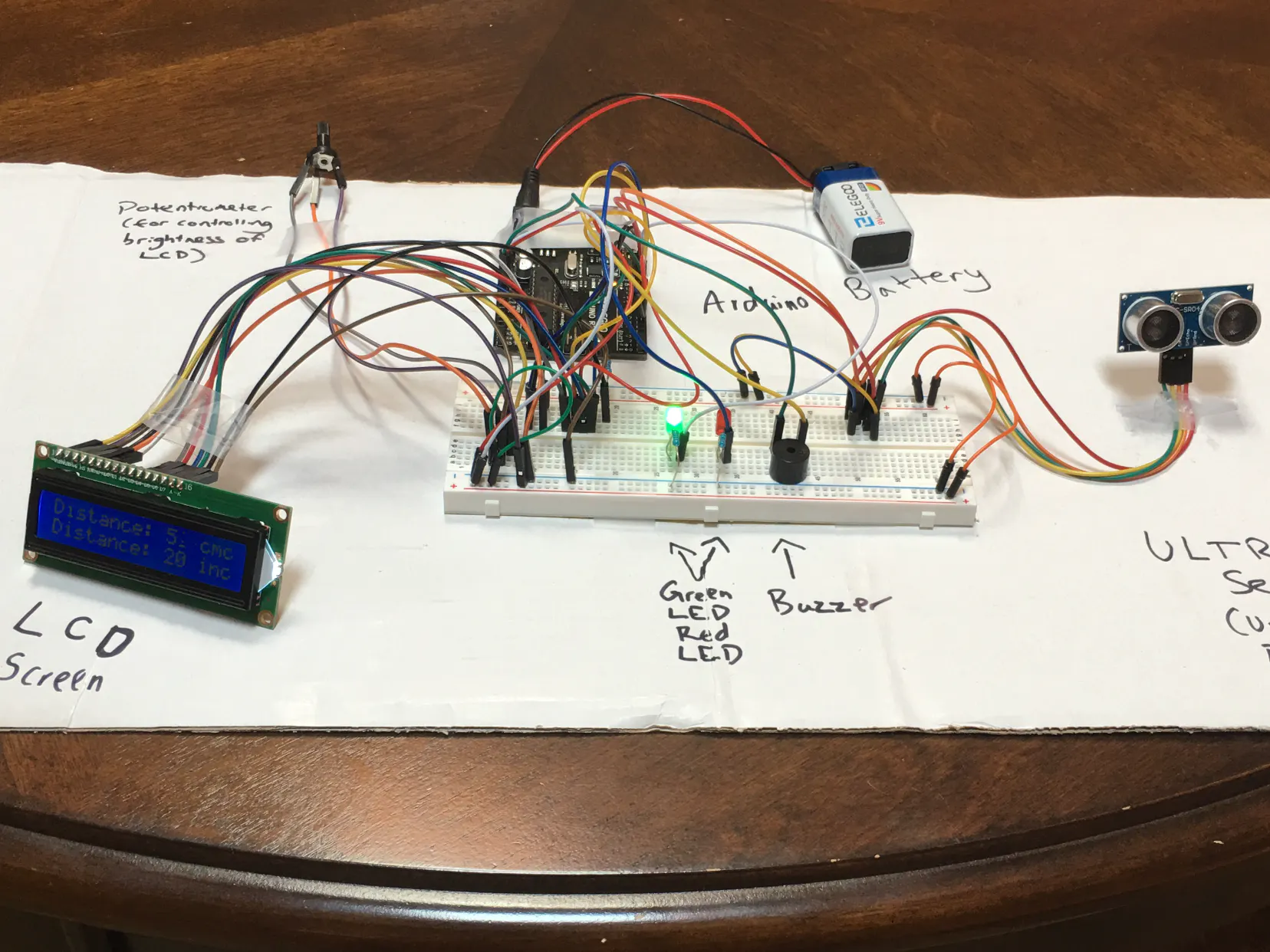## Things used in this project

### Hardware componentsUltrasonic Sensor - HC-SR04 (Generic)
×1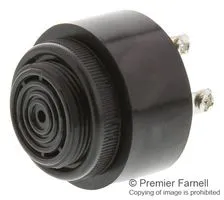Buzzer, Piezo
×1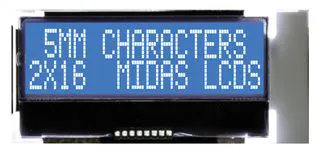Alphanumeric LCD, 16 x 2
×15 mm LED: Red
×1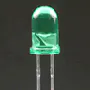5 mm LED: Green
×1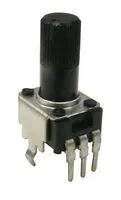Rotary Potentiometer, 10 kohm
×1

### Hand tools and fabrication machines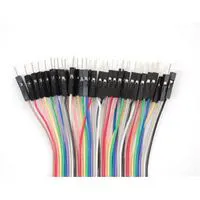Servo Motor, Premium Male/Male Jumper Wires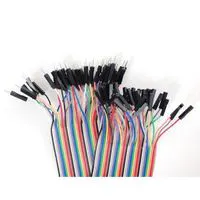Premium Female/Male Extension Jumper Wires, 40 x 6" (150mm)

## Schematics

### Arduino Security Alarm Schematic

This is my Arduino Security Alarm Schematic. Using a website called Tinkercad.com, I was able to create a schematic of my project, which is a Security Alarm made from an Arduino Board.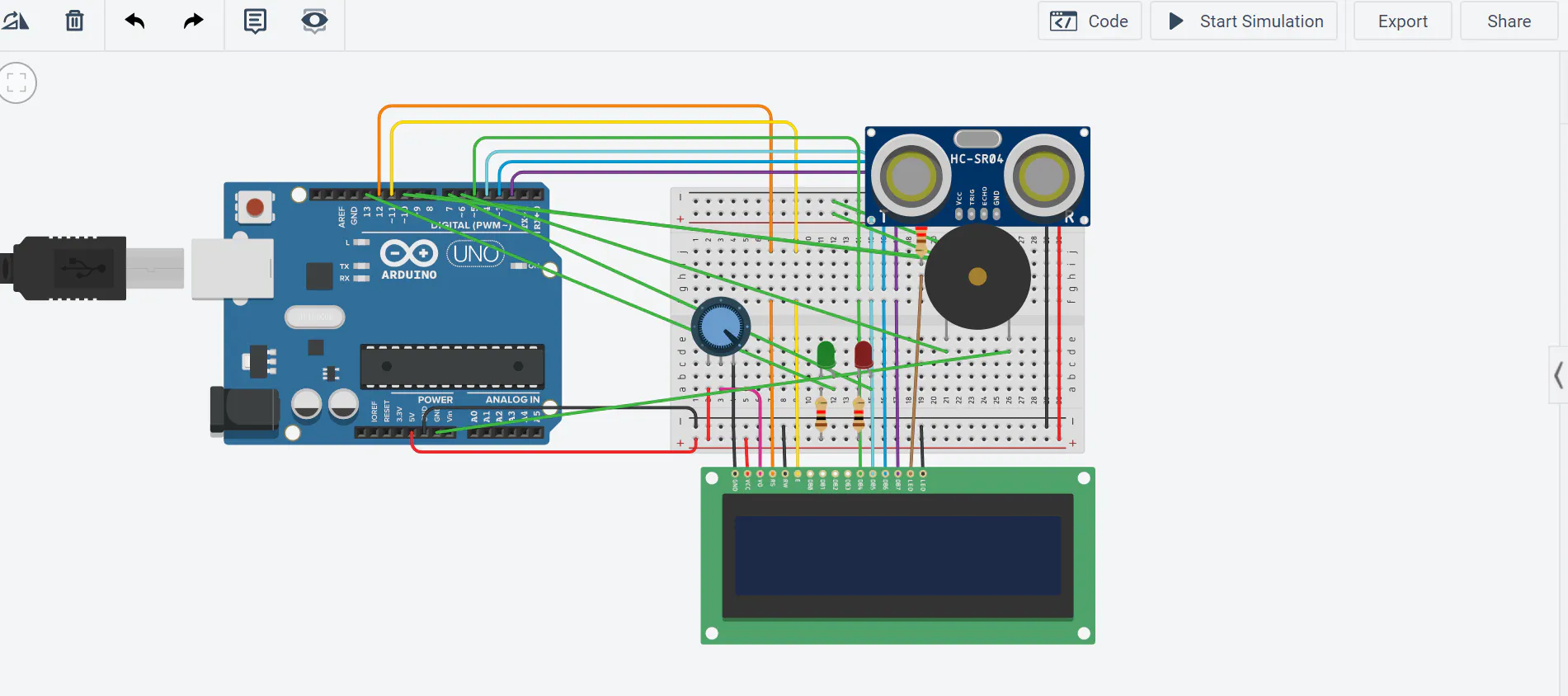## Code

### Arduino Security Alarm Code

C/C++
Using this code, using various commands, I was able to code each sensor so that if the distance is 40 centimeters or lower, then the buzzer will sound along with the appearance of a red LED light. However, if the distance is greater than 40 centimeters, then the buzzer will not sound and then a green LED will show. I also displayed the distance on an LCD screen (also connected to the Arduino) to give the owner an insight of how far away the person is using this code.
```// The LiquidCrystal library works with LCD displays that are compatible. For this project, I will be using a 16X2 LCD display.
#include <LiquidCrystal.h>
// Initialize the library with the numbers of the pins connected to the LCD Screen.
LiquidCrystal lcd (12, 11, 5, 4, 3, 2);

// Declaring the integers needed for this project
// Setting trigPin (Ultrasonic Sensor) to Pin 9
const int trigPin = 9;
// Setting echoPin (Ultrasonic Sensor) to Pin 10
const int echoPin = 10;
// Declaring duration for the distance formula later on
long duration;
// Declaring the distance in centimeters as well as the distance in inches
int distanceCm, distanceInch;
// Setting buzzerPin (buzzer) to Pin 6
const int buzzerPin = 6;
// Setting greenPin (LED) to pin 13
int greenPin = 13;
// Setting redPin (LED) to pin 7
int redPin = 7;

// Runs once
void setup() {

// Configuring buzzerPin as an OUTPUT value
pinMode (buzzerPin, OUTPUT);

// Set up the LCD's number of columns and rows
lcd.begin (16,2);
// Configuring trigPin as OUTPUT because the trigPin is sending out the sound signal to the object detected.
pinMode (trigPin, OUTPUT);
// Configuring echoPin as INPUT because the echoPin is recieving the signal from the object detected.
pinMode (echoPin, INPUT);

// Configuring the greenPin as OUTPUT.
pinMode (greenPin, OUTPUT);
// Configuring the redPin as OUTPUT.
pinMode (redPin, OUTPUT);
}

// Runs multiple times until the statement becomes false
void loop() {
// Sets the trigPin on a LOW state, as well as clearing the trigPin.
digitalWrite (trigPin, LOW);
// This will last for 2 microseconds.
delayMicroseconds(2);
// The trigPin will be on a HIGH state
digitalWrite (trigPin, HIGH);
// This will last for 10 microseconds for the Ultrasonic Sensor to sense the object.
delayMicroseconds(10);
// Then, after 10 microseconds, the trigPin will be set on a LOW state once more.
digitalWrite (trigPin, LOW);
// The echoPin will be set on a HIGH state to receive the signal and therefore be able to display the distance.
duration = pulseIn (echoPin, HIGH);
// Formula for calculating the distance in Centimeters.
distanceCm = duration*0.034/2;
// Formula for calculating the distance in inches.
distanceInch = duration*0.0133/2;
// Sets the LCD cursor to (0, 0), which is the top left of the LCD Screen
lcd.setCursor (0,0);
// The LCD screen will display "Distance: "
lcd.print ("Distance: ");
// The LCD screen will display the distance, in centimeters, which is being detected by the Ultrasonic Sensor.
lcd.print (distanceCm);
// The LCD screen will display " cm"
lcd.print (" cm");
// There is a delay of 0.01 seconds for the LCD screen to display the reading.
delay (10);
// The LCD cursor will move on to the next column.
lcd.setCursor (0,1);
// The LCD screen will display "Distance: "
lcd.print ("Distance: ");
// The LCD screen will display the distance, in inches, which is being detected by the Ultrasonic Sensor, as well.
lcd.print (distanceInch);
// The LCD screen will display " inch"
lcd.print (" inch");
// There is a delay of 0.01 seconds once more for the LCD screen to display the reading.
delay (10);

// This is an if-else statement. If the distance (in centimeters) is less than 40, then the red LED will turn on, the green LED will turn off, and the buzzer will sound.
if (distanceCm<=40){
digitalWrite (7, HIGH);
digitalWrite (13, LOW);
analogWrite (6, 255);
delay (0);
}
else{
// Otherwise, if the distance is greater than 40 centimeters (if the above statement is false), then the green LED will turn on, the red LED will turn off, and the buzzer will not sound.
digitalWrite (13, HIGH);
digitalWrite (7, LOW);
analogWrite (6, 0);
delay (0);
}
}

```

## Credits

### nithingarimella

0 projects • 0 followers